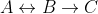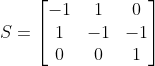A Python package to simulate biochemical systems using the Constraint-Based Simulation Algorithm

# Constraint-Based Simulation Algorithm (CBSA)

CBSA is a Python package for simulation of biochemical systems using the Constraint-Based Simulation Algorithm.## Installation

CBSA can be installed on your computer using pip.

Just run the following command:

python3 -m pip install cbsa --user --upgrade


## Usage

CBSA

### Simple reaction example

Consider the following reaction system:Using the Constrain-Based Modeling, the Stoichiometric matrix becomes:A sample code to simulate this system is:

import cbsa
import matplotlib.pyplot as plt
import numpy as np

S = [[-1, 1, 0],
[ 1,-1,-1],
[ 0, 0, 1]]

init_mols = [50,0,0]
k = [0.5,0.1,0.8]
max_dt = 0.1
total_sim_time = 10

sim = cbsa.ReactionSystem(S)
sim.setup()
sim.set_x(init_mols)
sim.set_k(k)
sim.set_max_dt(max_dt)

sim.setup_simulation()
sim.compute_simulation(total_sim_time)
sim_data = np.array(sim.simulation_data)

plt.plot(sim_data[:,0],sim_data[:,1],label="A")
plt.plot(sim_data[:,0],sim_data[:,2],label="B")
plt.plot(sim_data[:,0],sim_data[:,3],label="C")
plt.legend()
plt.show()## Project details

This version0.1.00.0.40.0.30.0.2

Uploaded source
Uploaded py3